## OSDM Demo Tutorial – Making of ‘Darkness – Oh Xee A Moron, Part 2′

Hi Folks,
Here is another Oldskool Demomaker Demo Tutorial of my popular production “Darkness, Oh Xee A Moron, Part 2“.

Also, all elements, such as 3D Objects, Images, Fonts and the Preference .INI Configuration file are also available for download here (Darkness.zip)

# Watch Video Capture

To get an idea how the final product looks like, without touching OSDM or downloading anything, watch the video capture that I created below.

Backup URL to Video on vimeo (http://www.vimeo.com/14096272).

Backup Links to Vimeo and YouTube, as well as additional download options (e.G. a Full Screen Version of the Demo) are available at my official demo release page.

# OSDM Settings Screen-By-Screen

## Main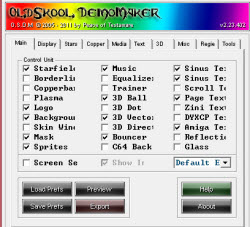Enabled Effects: Starfield Music Sinus Text 16 Sinus Text 32 3D Ball Page Text Logo Background 3D Vector Amiga Text Mask Bouncer Sprites Screen Setup: Off Default Export

## Display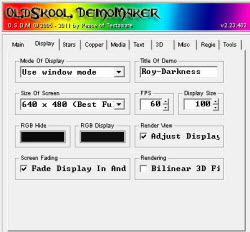Mode: Use window mode (full screen would shift 3d objects to invalid positions) Title: Roy-Darkness Size of Screen: 640×480 FPS: 60 (different value would mess up timing) Display Size: 100 RGB Hide & Display: Black Render View, Adjust Display: On Fade Display In And Out: On Bilinear 3D Filter: Off

## Stars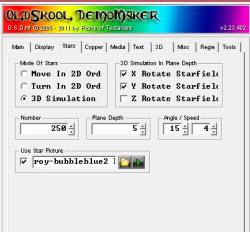Mode: 3D Simulation X Rotate Starfield: On Y Rotate Starfield: On Z Rotate Starfield: Off Number: 250 Plane Depth: 5 Angle: 15, Speed: 4 Use Star Picture: On (roy-bubbleblue2 bw.png)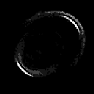## Logo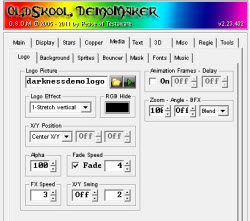Picture: darknessdemologo.png Animation: off Effect: 1-Stretch vertical RGB Hide: Black Zoom: 100, Angle: Off, BFX: Blend X/Y Position: Center X/Y Alpha: 100, Fade: On, Speed: 4 FX Speed: 3, X/Y Swing: Off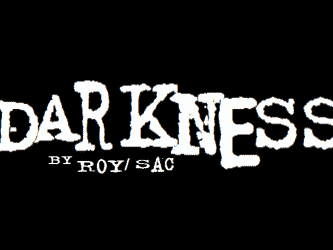## Background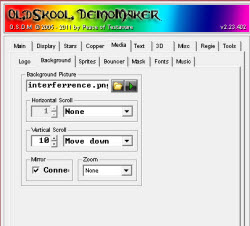Picture: interferrence.png Horizontal Scroll: None Vertical Scroll: 10, Move down Mirror: On Zoom: None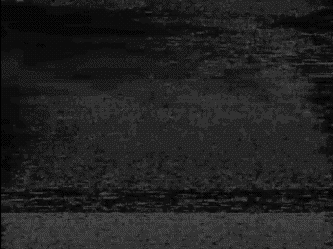## Bouncer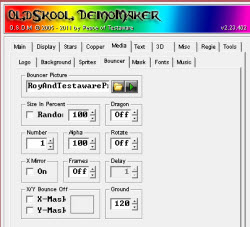Picture: RoyAndTestawareProd.png Size; Random: Off, 100%, Dragon: Off Number: 1, Alpha: 100, Rotate: Off X Mirror: Off, Frames: Off X-Mask & Y-Mask: Off Ground: 120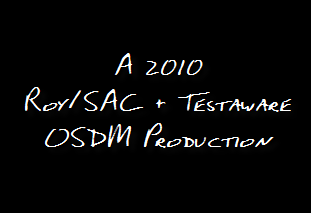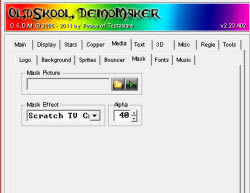Picture: None Mask Effect: Scratch TV Crumble Alpha: 40

## Fonts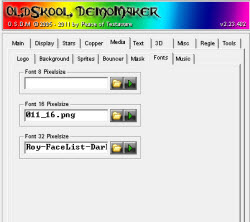16 Pixels: 011_16.png (for Sinus 16 Scroller)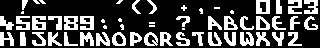32 Pixels: Roy-FaceList-Darkness-Font32x32-outlined.png (for Sinus 32 Scroller & Page Text)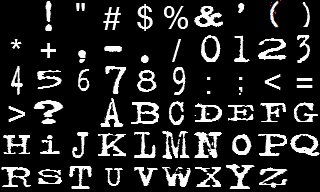## Music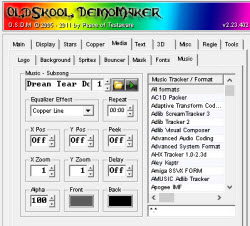Song: Drean Tear Down by Teque-Trauma.xm Subsong: 1

## Sprites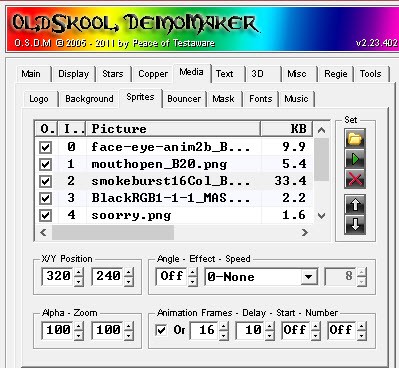Initial Sprites Settings

#NameX/Y-PosAngEffSpeed AlphaZoomAnimFrmsDelayStartNum
0face-eye-anim2b_B10.png320/240 Off 100100 On1090OffOff
1mouthopen_B20.png320/240 Off 100100 On2035OffOff
2smokeburst16Col_B16.png320/240 Off 100100 On1610
4soorry.png320/240 73-Move-left6 100100 Off
5darkness-note.png320/240 Off 100100 Off
6faceeyesopen2_B9.png320/180 Off 10090 On935
7visitroysaccom.png320/240 Off 100100 Off

 0 – face-eye-anim2b_B10.png 1 – mouthopen_B20.png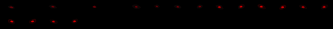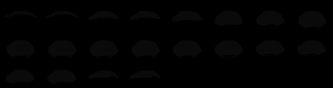2 – smokeburst16Col_B16.png 3 – BlackRGB1-1-1_MASK.png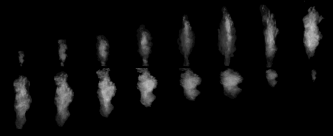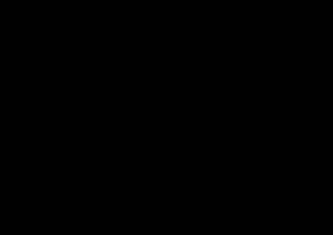4 – soorry.png 5 – darkness-note.png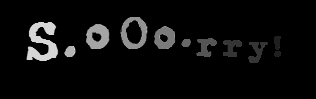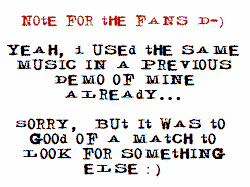6 – faceeyesopen2_B9.png 7 – visitroysaccom.png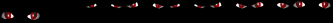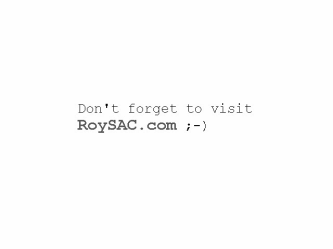## Sinus Text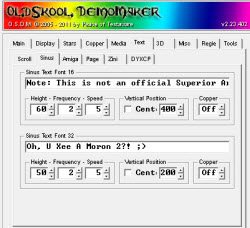Text Font 16: “Note: This is not an official Superior Art Creations production! None of my OSDM productions are. “ Height: 60; Frequency: 2; Speed: 5 Vertical Pos; Center: Off, 400 Copper: Off Text Font 32: “Oh, U Xee A Moron 2?! 😉 “ Height: 50; Frequency: 2; Speed: 5 Vertical Pos; Center: Off, 200 Copper: Off

## Amiga Text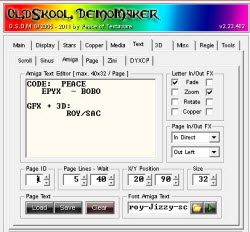Letter In/Out FX In: Fade Out: Zoom Page In FX: In Direct Page Out FX: Out Left Page Lines: 5; Wait: 40 X/Y Position: 20/90 Size: 32 Font Picture: roy-Jizzy-scenealphabet-font-OSDM-32×32-grayscale3.png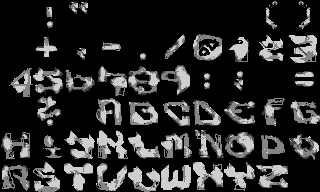Text Page 1 ```CODE: PEACE EPYX - BOBO GFX + 3D: ROY/SAC``` Page 2 ```[ [ [ [ [``` Page 3 ```MUSIC: "DREAM TEAR DOWN" BY TEQUE/TRAUMA``` Page 4 ```[ [ [ [ [```

## Page Text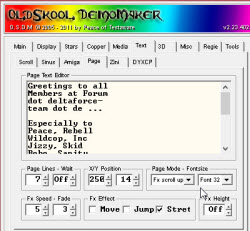Page Lines: 7, Wait: Off X/Y Position: 250/14 Page Mode: FX scroll up Font Size: 32 FX Speed: 5 Fade: 3 Fx Effect: Stretch Fx Height: Off Text: ```"Greetings to all Members at Forum dot deltaforce- team dot de ... Especially to Peace, Rebell Wildcop, Inc Jizzy, Skid Bobo, Sanity Dragon, Marie Genom, Synth Twilight and Janer. Cheers! Roy/SAC in 2010! Signing off. "```

## 3D Vector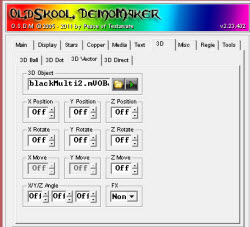Object: blackMulti2.mVOBJ X/Y/Z Position: Off X/Y/Z Rotate: Off X/Y/Z Move: Off X/Y/Z Angle: Off FX: None blackMulti2.mVOBJ consists of 4 individual vector objects 0…3 0 – Roy-Face20comb-Black-Closed.vobj 1 – sphere.vobj 2 – Roy-2TORUS-Black.vobj 3 – Roy-3TORUS-Black.vobj Build-in Tool to create Multi-Vector Object File from individual .VOBJ objects.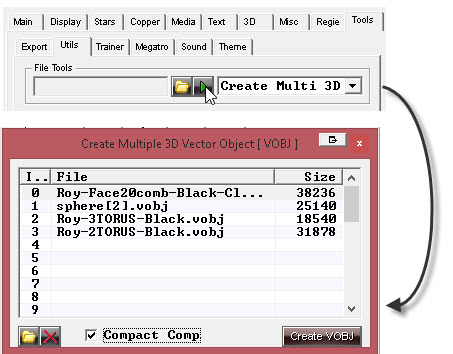0 – Roy-Face20comb-Black-Closed.vobj 1 – sphere.vobj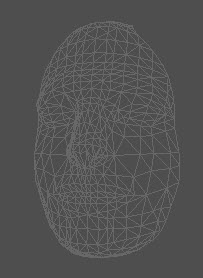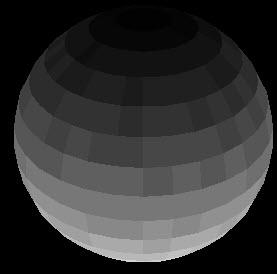2 – Roy-2TORUS-Black.vobj 3 – Roy-3TORUS-Black.vobj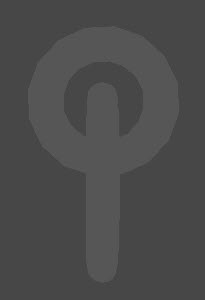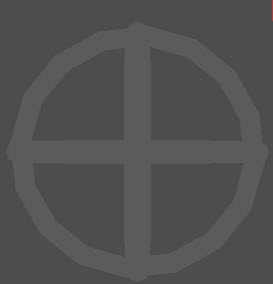## 3D Ball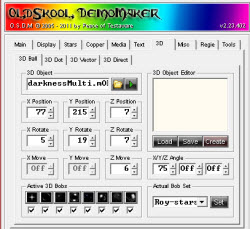Object: darknessMulti.mOBJ
X/Y/Z Position: 77/215/7
X/Y/Z Rotation: 5/19/7
X/Y/Z Move: Off/Off/6
X/Y/Z Angle: 75/Off/Off

Bobs Images

 0 1 2 3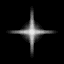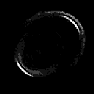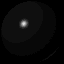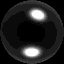4 5 6 7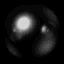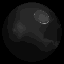darknessMulti.mOBJ consists of 6 individual 3D Ball objects 0-5

0 – rings3.obj
1 – rings4.obj
2 – roy-5rings-wobbler-vectormania.obj
3 – roy-CCU-Synth FullscreenCube.OBJ
4 – Roy-wall-1st Level Squared.obj
5 – Roy-wall-TowerObj.obj

Build-in Tool to create Multi-3DBall Object File from individual .OBJ objects.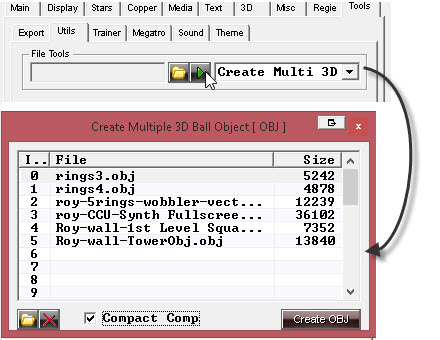0 – rings3.obj 1 – rings4.obj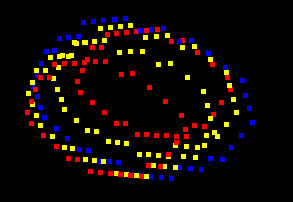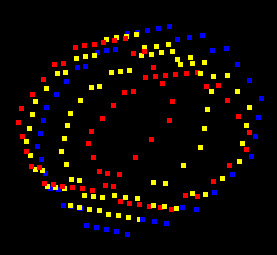2 – roy-5rings-wobbler-vectormania.obj 3 – roy-CCU-Synth FullscreenCube.OBJ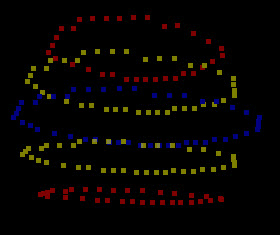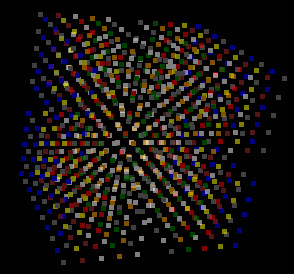4 – Roy-wall-1st Level Squared.obj 5 – Roy-wall-TowerObj.obj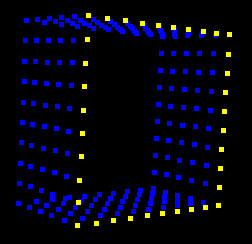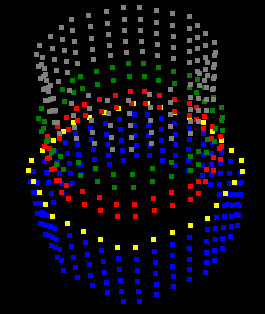## Script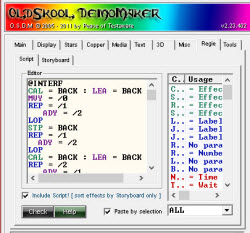Include Script!: On Script Source code shown further down

## Story BoardLine: Off, Fade: off, Speed: 1 Initial order from top to bottom. Only enabled effects are listed. D – Starfield G – 3D Vector Object I – 3D Ball Object L – Sinus Text 16 M – Sinus Text 32 N – Page Text H – Bouncer O – Mask X – Sprite W – Amiga Text J – Logo B – Background

## Export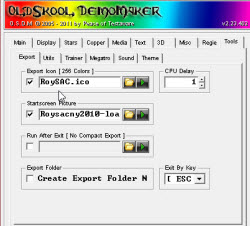Export Icon: RoySAC.ico
CPU Delay: 1 Exit by Key: [ ESC ] Startscreen Picture: Roysacny2010-loading2.png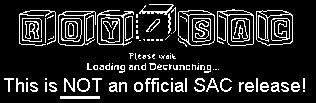# Script Code

```@INTERF
CAL = BACK : LEA = BACK
MVY = /0
REP = /1 : ADY = /2 : LOP
STP = BACK
REP = /1 : ADY = /2 : LOP
CAL = BACK : LEA = BACK
REP = /2 : ADY = /1 : LOP
STP = BACK
@END

;windowed/fs
;also see sprite init

@INIT3D
LEA = 3DVECTOR
IDN = /0
;Windowed
MVX = -600 : MVY = -350
;Full Screen
;MVX = 0 : ;MVY = -50
MVZ = -250
@END

;off for windowed
;enable for Full screen
@END

PSH
FPS = 60
CAL = MUSIC+SPRITE
LEA = SPRITE
IDN = 7 : MVA = 0
IDN = 6 : MVA = 0
;Windowed enable
;full screen disable
;next 1 line
IDN = 5 : MVA = 255
CAL = DISPLAY

;-----------------
;Init
;-----------------
LEA = SPRITE
IDN = 0 : MVA = 0 : ADY = -100 : ADX = 150
IDN = 1 : MVA = 0 : MVX = 500 : MVY = 380 : MVS = 900
IDN = 2 : MVA = 0 : MVX = 500 : MVY = 320 : ADW = -20
IDN = 3 : MVA = 0 : MVW = 640 : MVH = 480
IDN = 4 : MVA = 0 : ADX = 120 : ADY = 140

;------------------
NOP = 4
LEA = SPRITE : IDN = 5
REP = 120 : ADA = -2 : LOP
MVA = 0
STP = SPRITE

;----------------
;End Note Display
;--------------
CAL = 3DVECTOR
LEA = 3DVECTOR : IDN = 0

;--------------
;Init 3D Vect
;--------------
@INIT3D = 0

MAX = 0 : MAY = 0 : AAZ = 0
BFX = 2 : NOP = 2

REP = 100 : ADY = -2 : ADZ = -2 : LOP

@INTERF = 150,2,3

LEA = 3DVECTOR : IDN = 0

REP = 45 : AAX = 1 : ADY = -2 : ADZ = -2 : LOP
BFX = 4

@INTERF = 350,4,2

LEA = BOUNCE : MVX = 500 : MVY = 360
LEA = 3DVECTOR : IDN = 0
REP = 45 : AAX = 1 : ADY = -2 : ADZ = -2 : LOP
NOP = 1
MRY = -3 : NOP = 3
CAL = MASK : STP = BOUNCE
MRY = 4
REP = 150 : ADZ = 2 : ADX = -2 : LOP

@INTERF = 100,4,12

LEA = 3DVECTOR : IDN = 0
REP = 150 : ADZ = 2 : ADX = -2 : LOP
CPW = 240 : CPX = 2 : MRY = -4
REP = 150
IFX > -400 : ADX = 1
LOP
CAL = LOGO : CAL = MASK
MRY = 3 : BFX = 1 : NOP = 1
MRY = -3 : BFX = 4
REP = 150 :  ADZ = 3 : ADX = -3 : LOP
STP = LOGO : CAL = MASK
MRY = 0
REP = 90 : AAX = -1 : LOP
CPX = 0

;---------------
; Cloning End
;---------------
REP = 90 : AAX = 1 : LOP
@INTERF = 300,10,7
LEA = 3DVECTOR : IDN = 0
MRY = -3
REP = 200 : ADX = 2 : ADZ = -2 : LOP
MRY = 3 : NOP = 1

;---------------
;Eyes Blend in
;---------------
CAL = SPRITE
LEA = SPRITE : IDN = 0
REP = 200 : ADA = 1 : LOP
LEA = 3DVECTOR : IDN = 0 : MRY = 0

;---------------
;Mouth Open
;---------------
LEA = Sprite : IDN = 0 : MVS = 9999
IDN = 1 : MVS = 150 : MVA = 210 : NOP = 1 : MVS = 900

;-----------
;Smoke and Soorry
;------------
CAL = SINUS32 : LEA = SINUS32
MVZ = 105 : MVX = -325 : MVS = -3
LEA = SPRITE : IDN = 2
REP = 25
LOP
REP = 25
LOP
IDN = 1 : MVS = 150
REP = 200
IDN = 0 : ADA = -1
IDN = 1 : ADA = -1
IDN = 4 : ADA = 1
LOP
IDN = 0 : MVA = 0
IDN = 1 : MVA = 0
IDN = 4
REP = 20 : ADA = -10 : LOP
MVA = 0
STP = SPRITE
LEA = 3DVECTOR : IDN = 0
REP = 200 : ADX = 1 : LOP
STP = DISPLAY
REP = 100 : ADX = 2 : LOP
STP = SINUS32 : CAL = DISPLAY

;------------
;3D BALLS
; plus sphere
;------------
CAL = SPRITE+3DBALL
LEA = 3DBALL : IDN = 5
BFX = 1 : NOP = 1
@INTERF = 300,10,7
LEA = SPRITE : IDN = 3
REP = 150 : ADA = 1 : LOP

;--------------
;Sphere
;--------------
LEA = 3DVECTOR : IDN = 1
IDL = TRAINER
MVZ = 900 : ADY = 260
BFX = 1 : ARY = -30
MAX = 90 : MAY = -70
REP = 200
LOP
ARY = 15
LEA = 3DBALL : IDN = 5
ARY = -10
LEA = 3DVECTOR : IDN = 1
REP = 60
LOP
MRY = 3

;------------
;Object 5 - Tower
;--------------
LEA = 3DBALL : IDN = 5
ARY = -5 : NOP = 1
ARY = 5 : NOP = 1
ARY = 5 : NOP = 1
ARY = 5
CAL = LOGO
@INTERF = 400,15,5

;-------------
;Ball Bounce
;--------------
LEA = 3DVECTOR : IDN = 1
NOP = 3 : STP = LOGO
MRY = -15
REP = 50
LOP
MRY = -20 : MRX = 20
REP = 300
LOP
BFX = 4 :MRY = 15
REP = 200
LOP
NOP = 2 : MRY = -15

;---------------
;Credits
;---------------
CAL = AMIGATEXT
REP = 10 : ADX = -10 : LOP
REP = 60 : ADX = 10 : LOP
NOP = 2
REP = 60 : ADX = -10 : LOP
LEA = AMIGATEXT : IDN = 2
LEA = 3DVECTOR : IDN = 1
REP = 60 : ADX = 10 : LOP
NOP = 2
REP = 50 : ADX = -10 : LOP
NOP = 1
STP = AMIGATEXT

;--------------
REP = 100 : ADY = -6 : LOP
STP = 3DBALL
@INTERF = 150,5,11

;---------------
; 3 Torus
;---------------
CAL = STARS
LEA = 3DVECTOR : IDN = 2
IDL = TRAINER

;---------------

BFX = 4 : ADY = 1000
BFX = 4 : ADX = 50
REP = 75 : ADY = -5 : LOP

;-------------
;Greetings
;-------------
CAL = PAGE
MRX = 0
REP = 15
ARY = 1
IFR = 1 : IDN = 2
IFR = 3 : IDN = 2
IFR = 2 : IDN = 2
LOP
MRY = 0 : MRZ = 0 : BFX = 2
LEA = SPRITE : IDN = 3
REP = 50 : ADA = -3 : LOP
MVA = 0
@INTERF = 50,2,2
LEA = 3DVECTOR : IDN = 2
REP = 200
IFR = 8 : ARX = 2
LOP
MRZ = 10 : NOP = 3
BFX = 3 : ARX = 10
REP = 200
LOP
REP = 30
LOP
@INTERF = 100,4,8
LEA = 3DVECTOR : IDN = 2
REP = 150
LOP
BFX = 1
REP = 50
LOP
BFX = 4
@INTERF = 350,4,2
LEA = 3DVECTOR : IDN = 2
REP = 50
LOP
ARX = -10 : NOP = 4 : ARY = 20
REP = 300 : ADX = 4 : LOP
STP = DISPLAY : NOP = 1
STP = PAGE+STARS

;---------------
;3DBalls Part
;--------------
CAL = 3DBALL

;--------------
;Object 2 - Wobbler
;--------------
LEA = 3DBALL : IDN = 2
CAL = SPRITE
LEA = SPRITE : IDN = 3 : MVA = 150
CAL = DISPLAY
NOP = 4
REP = 75 : ADA = -2 : LOP
MVA = 0 : NOP = 2
@INTERF = 240,2,1

;--------------
;Object 1 - Rings 4
;--------------
LEA = 3DBALL : IDN = 1
REP = 150
LOP
REP = 180
LOP
REP = 100
LOP
REP = 80 : ADX = 1 : LOP
BFX = 2 : NOP = 2
@INTERF = 40,4,7

;--------------
;Object 0 - Rings 3
;--------------
LEA = 3DBALL : IDN = 0
NOP = 2
NOP = 1
LEA = SPRITE : IDN = 3
REP = 100 : ADA = 1 : LOP

;--------------
;Object 4 - Square
;--------------
LEA = 3DBALL : IDN = 4
BFX = 4 : MRX = -10
REP = 150 : AAZ = -2 : LOP
REP = 150 : AAZ = -2 : LOP
RGB = \$EEE : CLS = 2

;--------------
;Object 3 - Cube
;--------------
LEA = 3DBALL : IDN = 3
ADY = 100 : ARY = -10
REP = 20
LOP
ARY = -10 : NOP = 1
ARY = 5 : ARX = 5 : ARZ = 5 : NOP = 1
ARY = 5 : ARX = 5 : ARZ = 5 : NOP = 1
ARY = 5 : ARX = 5 : ARZ = 5 : NOP = 1
REP = 180
LOP
REP = 360
LOP
CAL = STARS

;-------------
;2 torus
;--------------
LEA = 3DVECTOR : IDN = 3
REP = 315
LOP
MRZ = 15 : MRX = 10 : MRY = 25
LEA = SPRITE : IDN = 3
REP = 100 : ADA = -1 : LOP
MVA = 0
LEA = 3DVECTOR : IDN = 3
REP = 150 : ADX = 2 : LOP
REP = 75
LOP
BFX = 1
REP = 75
LOP
REP = 75
LOP
BFX = 4
REP = 225 : ADY = -2 : LOP
CAL = LOGO : NOP = 4
STP = LOGO
REP = 300 : ADY = 4 : LOP

;------------
;Face Again
;------------
CAL = SINUS16
@INIT3D = 0
MRX = 0 : MRY = 0 : MRZ = 0 : ADX = 400 : ADY = 0
MAY = 0 : MAZ = 0 : MAX = 90 : MRY = 2
REP = 200 : ADX = -2 : LOP
MRY = -2 : NOP = 3 : MRY = 0

;-------------
; Eye Animation
;-------------
CAL = SPRITE
LEA = SPRITE : IDN = 6 : MVA = 255
STP = DISPLAY+SINUS16
LEA = 3DVECTOR : IDN = 0 : BFX = 1 : NOP = 1
STP = STARS+3DVECTOR

;----------------
; Vist RoySAC.Com
;----------------
CAL = DISPLAY
LEA = SPRITE
REP = 54
IDN = 6 : ADA = -4
IDN = 7 : ADA = 4
LOP
IDN = 6 : MVA = 0
IDN = 7 : MVA = 255
NOP = 2

;-------------
;-------------
REP = 99 : ADV = -1 : LOP
NOP = 1

ESC : END
```

# Closing Notes

For more OSDM tutorials, tools, demos, intros and resources; visit the OSDM Section on my web site.

Cheers!

Carsten aka Roy/SAC

## Post details

Categories: EDUCATION OSDM TUTORIAL
Tags:
Published on: September 11, 2014